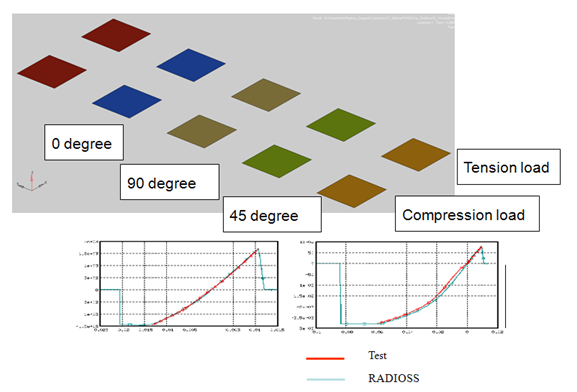# Composites

5.1 Composite non linear behavior in Radioss for crash and impact analysis

Two main material models represent in detail the behavior of composites:

• Tsai Wu,
• CRASURV  based on Tsai-Wu with yield surfaces for hardening and strain rate effect

They can be associated to shell, thick shells & solid elements

• Tsai-Wu yield criterion formulation: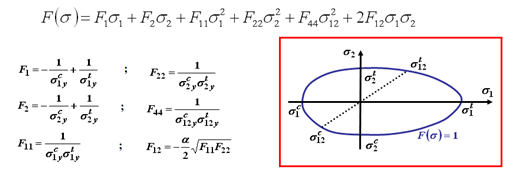The model is defined using seven parameters:

• Tension and compression yield stresses with respect to directions 1 & 2
• Shear yield stresses and reduction factorα (safety margin for the ellipse, not necessary uniform, by default α = 0 )

The Tsai-Wu coefficients are automatically calculated by Radioss  from prescribe critical stress values:

• Crasurv is a variant of Tsai-Wu, where coefficients depend upon plastic work

(Energy absorbed by the composite)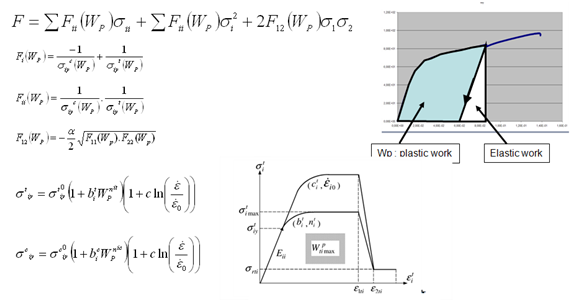This law allows identifying independently each direction and gives a good correlation for each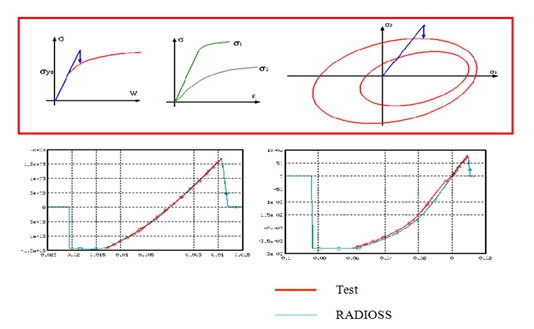• Remark on the Chang-Chang model proposed:

Chang-Chang law incorporated in RADIOSS is a combination of the standard Tsai-Wu elastic-plastic law and modified Chang-Chang failure criteria. The effects of damage are taken into account by decreasing stress components using a relaxation technique to avoid numerical instabilities.

5.2  Tensile rupture

Tensile rupture in composite is difficult because it is, most of the time. brittle

Deleting the element at the straight value of rupture strain will lead to numerical instabilities. So it is therefore important to introduce a relaxation slope to smooth the energy release due to failure.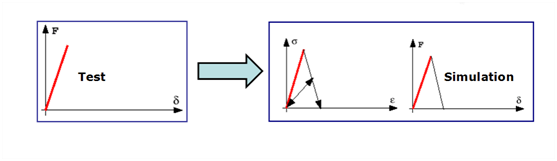No Discharge SlopeWith discharge slope

We can notice that without discharge slope, a huge amount of elements is deleted which is not realistic. It’s coming from the “shock” due to the deletion at high energy level, without relaxation of it.

5.3  Rupture failure Criteria

1. Chang-Chang
2. Hashin
3. Puck
5. Ladeveze / Allix for delamination

5.3.1        Chang-Chang

Six material parameters are used in the failure criteria:

S1 =  Longitudinal tensile strength

S2 = Transverse tensile strength

S12 =  Shear strength

C1 =  Longitudinal compressive strength

C2 =  Transverse compressive strength

β = Shear scaling factor.

(1 = fiber direction)

The failure criterion for fiber breakage is written as: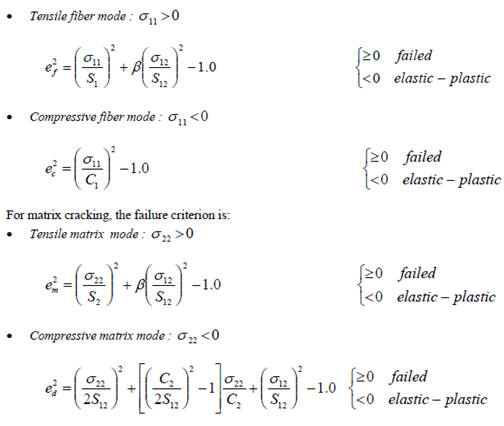5.3.2        Hashin

 It’s a unidirectional lamina model:

Tensile/shear fiber mode: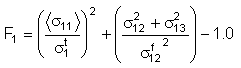Compression fiber mode: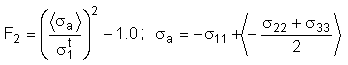Crush mode: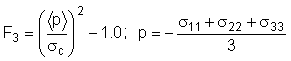Failure matrix mode: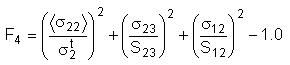Delamination mode: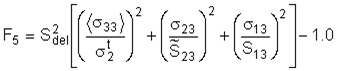5.3.3        Puck

 The failure mode criteria is written as:
• Tensile fiber failure mode: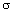11  > 0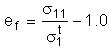• Compressive fiber failure mode:11  < 0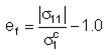• Inter fiber failure:
• Mode A  if22 >  0: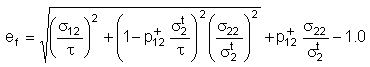• Mode C  if22 <  0: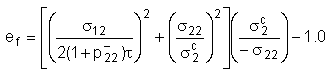• Mode B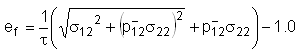It’s a combination of the Hashin and Puck models.

5.4  Material Characterizations

Non- linear properties for orthotropic material have to be determined through 5 sets of data:

• Tension on 0 degrees
• Compression in 0 degrees
• Traction in 90 degrees
• Compression in 90 degrees
• Traction/Compression 45 degrees

ASTM  testing procedures are recommended::

• Tensile testing of Polymers matrix composite Materials ASTM D3039, D3039M
• Compressive Properties of Polymer Matrix Composite Materials by shear loadings ASTM D3410, D3410M
• In-plane shear response of Polymer Matrix Composite Materials by tensile test of a ± 45° Laminate ASTM D3518, D3518M

Curve fitting process:

Initial data :     stress-strain curve from uniaxial compression

(or tension) S1(E1)

Step 1:             Scale the curve to get shear stress as function

of the shear angle

Υ12=2*E1 (this is an approximate equation)

τ12= ½ S1

Step 2:             Determine the initial slope = G12

Calculate elastic energy =

Calculate plastic work =

Step 3:             Represent τ 12 as function of the plastic work

A simple one-shell model is recommended to verify the material card data